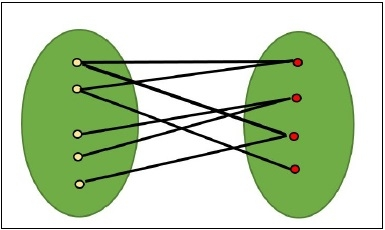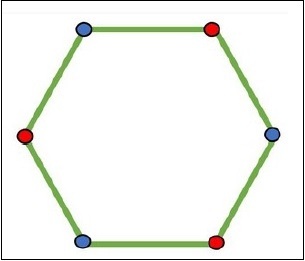# How to find if a graph is Bipartite?

A graph is said to be a bipartite graph, when vertices of that graph can be divided into two independent sets such that every edge in the graph is either start from the first set and ended in the second set, or starts from the second set, connected to the first set, in other words, we can say that no edge can found in the same set.Checking of a bipartite graph is possible by using the vertex coloring. When a vertex is in the same set, it has the same color, for another set, the color will change.## Input and Output

Input:
0 1 1 1 0 0
1 0 0 1 1 0
1 0 0 1 0 1
1 1 1 0 1 1
0 1 0 1 0 1
0 0 1 1 1 0

Output:
The graph is bipartite.

## Algorithm

isBipartite(source)

Input − The source vertex.
Output: True when the graph is bipartite.

Begin
define an empty queue qu, and a color list coloArray
initially any node is not colored with any color
color the source vertex as color red
when qu is not empty, do
remove item from the qu and take in u
if there is any self-loop, then
return false
for all vertices v, which is connected with u, do
if v has no color, then
if colorArray[u] = red, then
colorArray[v] := blue
else if colorArray[u] = blue, then
colorArray[v] := red
if colorArray[v] = colorArray[u], then
return false
done
done
return true
End   

## Example

#include<iostream>
#include<string>
#include<queue>
#define NODE 6
using namespace std;

/*int graph[NODE][NODE] = {
{0, 1, 1, 1, 0, 0},
{1, 0, 0, 1, 1, 0},
{1, 0, 0, 1, 0, 1},
{1, 1, 1, 0, 1, 1},
{0, 1, 0, 1, 0, 1},
{0, 0, 1, 1, 1, 0}
}; */

int graph[NODE][NODE] = {
{0, 1, 0, 0, 0, 1},
{1, 0, 1, 0, 0, 0},
{0, 1, 0, 1, 0, 0},
{0, 0, 1, 0, 1, 0},
{0, 0, 0, 1, 0, 1},
{1, 0, 0, 0, 1, 0}
};

bool isBipartite(int source) {
queue<int> qu;
string colorArray[NODE];

for(int i = 0; i< NODE; i++)
colorArray[i] = "No Color";    //initially no color is set for all vertices
colorArray[source] = "Red";    //assign red with the source vertex
qu.push(source);             //add source into the queue.

while(!qu.empty()) {
int u = qu.front();
qu.pop();
if(graph[u][u] == 1)    //there is a self loop
return false;

for(int v = 0; v < NODE; v++) {
if(graph[u][v] != 0 && colorArray[v] == "No Color") {
if(colorArray[u] == "Red")       //assign adjacent list with alternate color
colorArray[v] = "Blue";
else if(colorArray[u] == "Blue")
colorArray[v] = "Red";
} else if(graph[u][v] != 0 && colorArray[v] == colorArray[u]) {
return false;       //when u and adjacent are of same color.
}
}
}
return true;
}

int main() {
bool check;
check = isBipartite(0);

if(check)
cout << "The graph is bipartite." << endl;
else
cout << "The graph is not bipartite." << endl;
}   

## Output

The graph is bipartite.